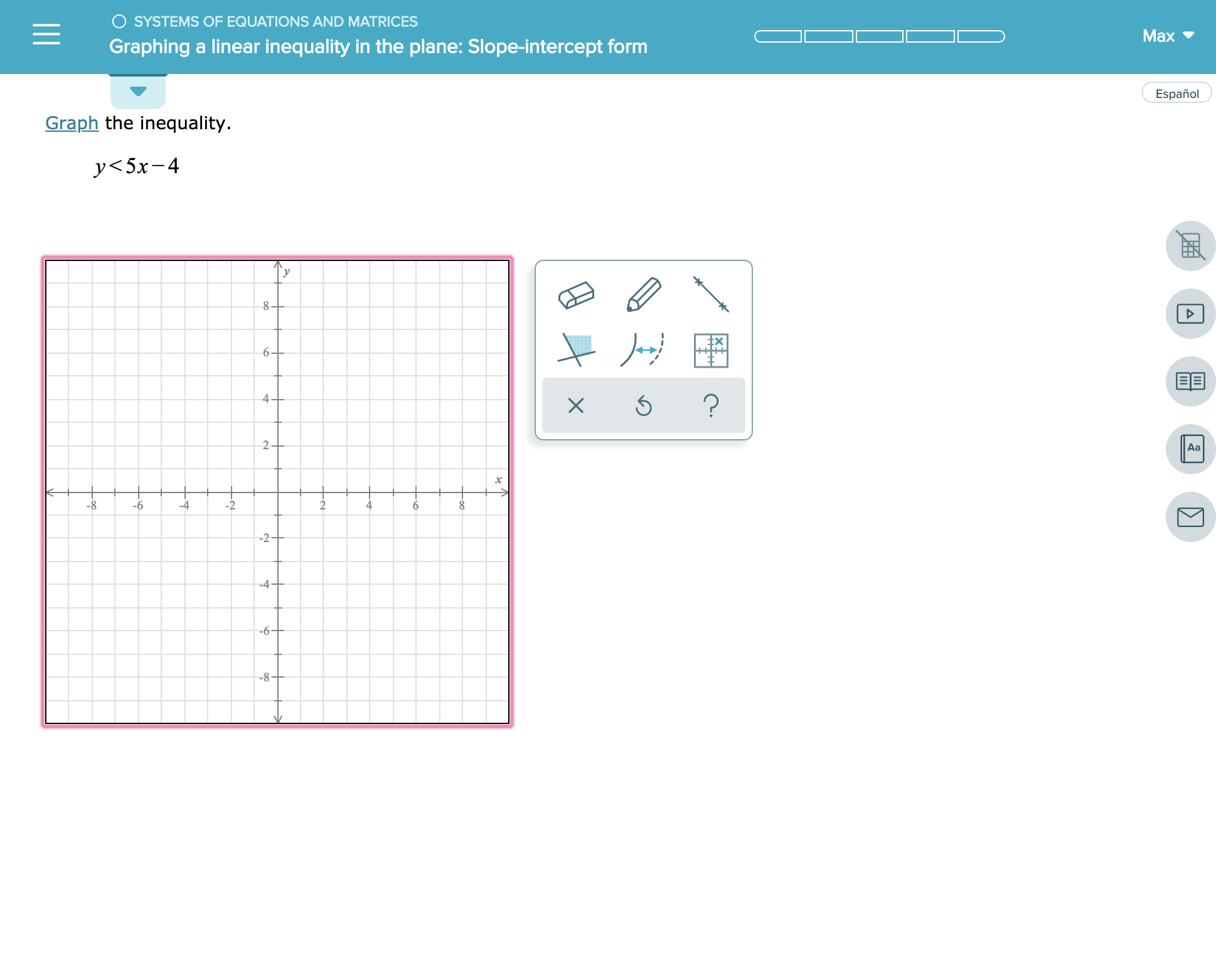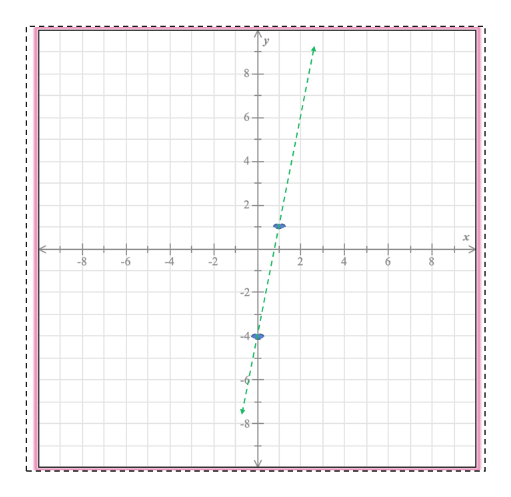SYSTEMS OF EQUATIONS AND MATRICESMaxGraphing a linear inequality in the plane: Slope-intercept formEspañolGraph the inequality.у

Question

see attachmenthelp_outlineImage TranscriptioncloseSYSTEMS OF EQUATIONS AND MATRICES Max Graphing a linear inequality in the plane: Slope-intercept form Español Graph the inequality. у<5х-4 6- 4+ 2 Aa -2 -8 4 2 -6 6 -2- -4- -6 -8- X fullscreen
Step 1

y < 5x - 4

As a first step let\'s plot the straight line y = 5x - 4

We need just two points to plot the line. Easiest way to get the two points is:

When x = 0, y = -4

When x = 1, y = 1

Hence, the two points are (0, -4) and (1, 1)

Please see the white board. The green line is the plot of y = 5x - 4help_outlineImage Transcriptionclose8 6 4- 2+ 8 -6 4 2 2 4 6 -2- -6 -8+ fullscreen
Step 2

Now test an arbitrary point say (0, 0)

y < 5x - 4 = 5 x 0 - 4 = -4

Hence, ...

Want to see the full answer?

See Solution

Want to see this answer and more?

Our solutions are written by experts, many with advanced degrees, and available 24/7

See Solution
Tagged in

Calculus